# Euler’s Method Using MATLAB

May 23, 2020

By  MATLAB Helper

lang: en_US

## Introduction and History

Leonhard Euler, one of the most prolific mathematicians of the 18th Century, invented the Euler’s method. To those unaware, he was a very eminent mathematician, and his influence on the world of mathematics is something one cannot oversee.

-Pierre-Simon Laplace

Above quote is rightly describes the importance of how great the work of Euler was in the field of mathematics. He has written about 500 books and papers during his lifetime. Euler’s method is not the just one concept named after him. There are many other concepts named after him; Euler’s constant, Euler line of triangle, Euler’s equation of motion, Eulerian graphs, to name a few. He has worked on a variety of area including astronomy, logarithms, calculus, the motion of the moon and plenty more. It is not surprising that he is such a high figure of influence in the world of mathematics. His concepts made mathematical applications so understandable for everyone today. Euler’s method is one such concept which we are going to implement using MATLAB in this blog!

## A Quick Understanding of Euler’s Method

Euler’s method is a technique to solve first order initial value problems (IVP), numerically. The standard form of equation for Euler’s method is given aswhere y(x0) = y0 is the initial value. We need to find the value of y at point ‘n’ i.e. y(xn).

Right now, we know only one point (x0, y0). The blue graph below is the ‘Exact’ (Solution Curve in Diagram) and the red / tangent line is the Euler’s method. Our aim is to find the set of points along with this blue graph. Let us see the steps to find the solution.

• We use the tangent line (red line) as an approximation at the only known point to us, (x0, y0).
• We move a short distance ahead on this red line which is close to our true solution (x1, y1). This short distance is the step size and denoted by ‘h’.
• For better approximate values, keep the value of h as small as possible.
• We now use the point (x1, y1) to plot the point (x2, y2) in the similar fashion.
Repeat the steps until you have reached the final point (xn, yn).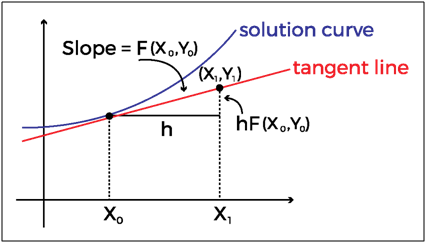Image 1: Euler's Approximation

We will use this method to find the solution to a differential equation of a basic RC circuit. Let us see how!

## Example

We’re going to approximate the charge on the capacitor in the circuit given below and plot the graph using MATLAB. Then compare the Exact and the Euler’s graphs.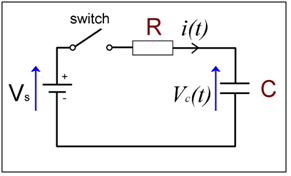Image 2: RC Circuit

#### Differential Equation of the Given RC Circuit

In the circuit, R represents resistance in Ohms, C is capacitance in Farads, i(t) is current through the resistor measured in Amperes and the capacitor has the voltage drop Q/C. Q(t) is the charge on capacitor at time t. When the switch is closed at time t = 0, by applying Kirchhoff’s law we get the equation: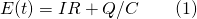A few math steps will give us the equation in the form of Euler’s method as below:We’re going to use equation (2) to write the code and plot the approximation graph in MATLAB

#### Given Parameters

• E = 200 V. R = 1500 Ω. C = 0.0025F.
• Initial Condition: Q = 0 at t = 0
• Find Q at t = 1.

#### Steps to MATLAB Code

1. Defining the equation 2 in MATLAB
2.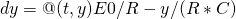;
3. Set the initial and final time values a = 0, b = 1 and y0 = 0;
4. Number of steps N = 10
5. Therefore:  Step size h = (b-a)/N
6. Run the for loop till N number of steps
7.(increment t by h)
8. Plot (t, y) – value of Q against time t
9. Repeat the same steps for True value with the following equation. This is the first order differential through mathematical analysis.
10.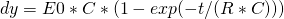;
Verify the plot with the figure below!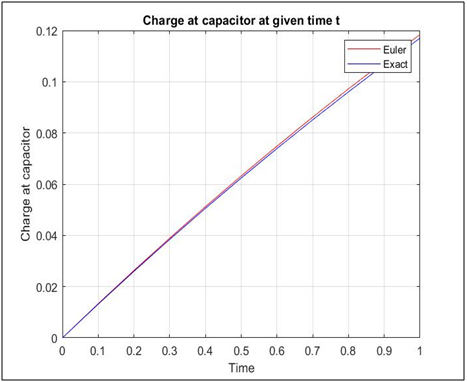Fig 3: MATLAB Simulation Result

You may also plot a table to display value of Q at time ‘t’ for N number of steps. To do so you may use the function table in MATLAB.  We’ve shown the values for both the methods below!

## Exact & EulerValues

 Steps Time Charge at Capacitor – Exact Charge at Capacitor - Euler 1 0 0 0 2 0.1 0.013157 0.013333 3 0.2 0.025968 0.026311 4 0.3 0.038442 0.038943 5 0.4 0.050587 0.051238 6 0.5 0.062413 0.063205 7 0.6 0.073928 0.074853 8 0.7 0.08514 0.08619 9 0.8 0.096057 0.097225 10 0.9 0.10669 0.10797 11 1 0.11704 0.11842

## Conclusion

The graph and tables above show how accurately we could calculate the charge at the capacitor in above RC circuit. Hence, we could safely use Euler’s method to solve the first order differential equations even in the problems where we do not know the exact solution.

Ready to take your MATLAB skills to the next level? Look no further! At MATLAB Helper, we've got you covered. From free community support to expert help and training, we've got all the resources you need to become a pro in no time. If you have any questions or queries, don't hesitate to reach out to us. Simply post a comment below or send us an email at [email protected].

And don't forget to connect with us on LinkedIn, Facebook, and Subscribe to our YouTube Channel! We're always sharing helpful tips and updates, so you can stay up-to-date on everything related to MATLAB. Plus, if you spot any bugs or errors on our website, just let us know and we'll make sure to fix it ASAP.

Ready to get started? Book your expert help with Research Assistance plan today and get personalized assistance tailored to your needs. Or, if you're looking for more comprehensive training, join one of our training modules and get hands-on experience with the latest techniques and technologies. The choice is yours – start learning and growing with MATLAB Helper today!

Education is our future. MATLAB is our feature. Happy MATLABing!

#### MATLAB Helper

MATLAB Helper ® is an organization providing programming expertise with assistance to students, professionals, researchers & and corporate.

•tanu jain says:

eulers method by matlab has advantage for research work…

•tanu jain says:

#### MATLAB Helper ®

Use Website Chat or WhatsApp at +91-8104622179